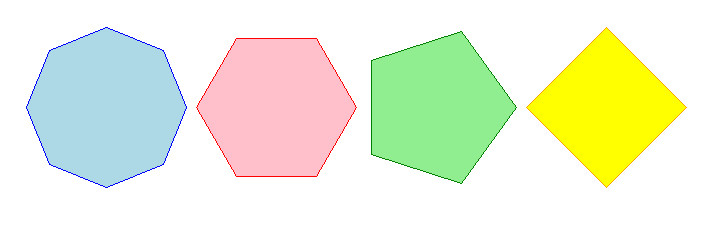# 11. Turtle regular polygons

The code below draws regular polygons; that is, polygons with equal angles and each sides.

## 11.1. Turtle Circles as polygons

Use the turtle syntax for drawing circles to draw regular polygons by specifying a given steps number:
radius - radius; a positive number draws anticlockwise, a negative number draws clockwise.
extent - an angle; a number (or None for whole circle), which determines how many degrees of the circle is drawn; default None
steps - an integer (or None for a circle) which allows polygons to be drawn; default None
To draw a triangle, use `steps=3`
To draw a square, use `steps=4`
To draw a hexagon, use `steps=6`

## 11.2. Regular polygons at a specified location

Adding a starting position, the centre of the regular polygon, will provide some convenience.
The `draw_centered_circle` syntax is below:
draw_centered_regular_polygon(t, centre=(0, 0), radius=20, sides=4, penw=1, penc='black', fillc=None)
t - the turtle object to draw the regular polygon
centre - start position; default (0, 0)
sides - the number of sides; default 4
penw - the pen width; default 1
penc - the pen color; a colorstring or a numeric color tuple (r, g, b,); default “black”
fillc - the fill color; a colorstring or a numeric color tuple (r, g, b,); default None
The `draw_centered_regular_polygon` definition code is below.
The code moves the turtle to the given centre, sets the angle to 0, moves forward the radius and sets the angle to 90, then draws the regular polygon.
If there is a fill color given, then `begin_fill` and `end_fill` need to be used either side of the drawing.

Code Completion: draw_centered_regular_polygon definition

Complete the code for the `draw_centered_regular_polygon` definition by replacing the “XXX”s.

```def draw_centered_regular_polygon(t, centre=(0, 0), radius=10, sides=4,
penw=1, penc="black", fillc=None):
t.pu()
t.goto(XXX)
t.seth(0)
t.fd(XXX)
t.seth(90)
t.pensize(penw)
t.pencolor(penc)
t.pd()
if fillc is not None:
t.fillcolor(fillc)
t.begin_fill()
t.circle(XXX, steps=XXX)
if fillc is not None:
t.end_fill()
```

Completed code for the `draw_centered_regular_polygon` definition.

```def draw_centered_regular_polygon(t, centre=(0, 0), radius=10, sides=4,
penw=1, penc="black", fillc=None):
t.pu()
t.goto(centre)
t.seth(0)
t.seth(90)
t.pensize(penw)
t.pencolor(penc)
t.pd()
if fillc is not None:
t.fillcolor(fillc)
t.begin_fill()
if fillc is not None:
t.end_fill()
```

## 11.3. Simple regular polygons

1. Use the definition provided above to draw polyogns of 3, 4, 5,and 6 sides.Use the definition provided above to draw polygons of 3, 4, 5,and 6 sides.

```import turtle

def draw_centered_regular_polygon(t, centre=(0, 0), radius=10, sides=4,
penw=1, penc="black", fillc=None):
t.pu()
t.goto(centre)
t.seth(0)
t.seth(90)
t.pensize(penw)
t.pencolor(penc)
t.pd()
if fillc is not None:
t.fillcolor(fillc)
t.begin_fill()
if fillc is not None:
t.end_fill()

s = turtle.Screen()
s.bgcolor("white")
s.title("draw_centered_regular_polygon")
s.setup(width=800, height=600, startx=0, starty=0)

t = turtle.Turtle()
t.speed(0)
t.ht()

centres = [(-250, 0),(-80, 0),(80, 0),(250, 0)]
radii = [80, 80, 80, 80]
polysides = [8, 6, 5, 4]
pensizes =  [1, 1, 1, 1]
pencolors = ["blue", "red", "green", "orange"]
fillcolors = ["light blue", "pink", "light green", "yellow"]